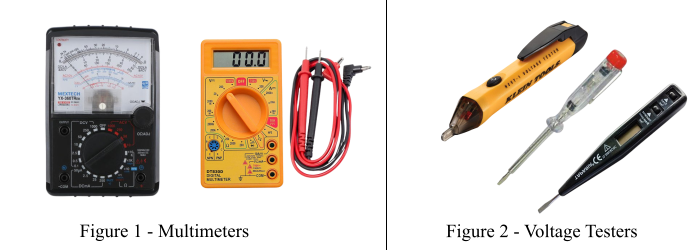# Difference Between Multimeter and Voltage Tester

Multimeters and voltage testers are both testing and measuring devices that are used to measure a wide range of electrical quantities. Go through this article to get a basic understanding of how multimeters and voltage testers work and how exactly they are different from each other.## What is a Multimeter?

A multimeter is a standard measuring instrument that is used for measuring multiple electrical quantities in an electric circuit. With a typical multimeter, one can measure AC voltage and current, DC voltage and current, resistance, continuity, and other values.

Basically, a multimeter is a combination of a DC voltmeter, AC voltmeter, DC ammeter, AC ammeter and ohmmeter. The multimeter is one of the important measuring instrument which is preferred by electricians for troubleshooting the problems in electrical appliances, motors, circuits, etc.

### Types of Multimeters

Based on the design and method of measurement and display, multimeters are classified into two types as −

• Analog Multimeter
• Digital Multimeter

An analog multimeter is the type of multimeter based around the use of a moving coil meter. It consists of a display of analog form that uses the deflection of a pointer to indicate the level of the measurement. The analog multimeters are mainly used to measure electrical quantities like voltage, current, resistance, frequency and power, etc.

A digital multimeter (DMM) is a measuring device which can also be used to measure electrical quantities such as voltage, current and resistance. In a digital multimeter, the measured value of the quantity is displayed on a digital screen.

Both analog and digital multimeters have their own advantages. Thus, one can choose an instrument based upon the requirements.

## What is Voltage Tester?

A voltage tester is a simple electrical testing instrument which is used to check the presence and absence of the electric charge or electric potential in an electric circuit. The voltage tester is also known as voltage detector. Basically, it is a pen shaped testing device. A typical voltage tester consists of a small neon bulb with two insulated wires attached to the bottom of the bulb housing. Each wire ends in a test probe made of metal.

A voltage tester is always used with the current turn-on to test whether there is current flowing through a wire and to test for proper grounding. The voltage tester can also be used to test whether adequate voltage is present in a wire.

In order to use a voltage tester, one probe is attached to the wire and the other probe goes to the neutral. If the wire or component is receiving electricity, the neon bulb will glow. If it does not glow, then there is a trouble at this point.

## Difference between Multimeter and Voltage Tester

The following table shows the key differences between multimeter and voltage tester.

Parameter
Multimeter
Voltage Tester
Definition
A multimeter is a standard measuring instrument that is used for measuring multiple electrical quantities in an electric circuit.
A voltage tester is a simple electrical testing instrument which is used to check the presence and absence of the electric charge or electric potential in an electric circuit.
Function
A single multimeter can function as a voltmeter, ammeter, ohmmeter, frequency meter, etc.
A voltage tester functions only as a detector for electricity in an electric circuit or component.
Working
A multimeter has two leads with a metal probe on each end. When these two probes are connected in a circuit, the multimeter injects a small current into the circuit and then taking reading of the measured quantity.
A voltage tester also has two leads with a metal probe on each end. These metal probes are connected in the circuit, where one probe touches the live wire and the other probe goes to the neutral. If there is electricity (or current) present in the circuit, the neon bulb will glow.
Value of measurement
Multimeter provides the value of measurement in the standard units.
Voltage tester only detects the presence and absence of electric charge and does not provide its value.
Design
The design and construction of a multimeter is complex as it composes multiple instruments.
The voltage tester is simple in design.
Size and shape
A multimeter is relatively bigger in size and shaped as a palmtop (handheld).
The voltage tester is compact in size and is of pen-shaped.
Type
Based on the design and method of measurement and display, the multimeters are of two types viz. analog multimeter and digital multimeter.
The voltage testers are of following types viz. neon voltage tester, digit voltage tester, non-contact voltage tester, etc.
Cost
Multimeters are costlier than a voltage tester.
The cost is of a voltage tester is comparatively low.
Applications
Multimeters are widely used in electrical testing and troubleshooting. They are widely used to measure the value of electrical quantities such as voltage, current, resistance, etc.
Voltage tester is also used in electrical and electronic testing and it is mainly employed to determine the presence and absence of electricity in an electric wire or circuit.

## Conclusion

From the above discussion, it can be concluded that there are several differences between a multimeter and a voltage tester. Voltage testers are easier to use than a multimeter. Voltage testers detect the presence or absence of the voltage in a circuit without having a touch with the wire. However, voltage testers are not accurate at all the times because they do not provide a value of the measured quantity. On the other hand, multimeters are efficient, multipurpose and accurate measuring instruments. Multimeters can detect various electrical quantities (mainly voltage, current, and resistance) in a circuit.